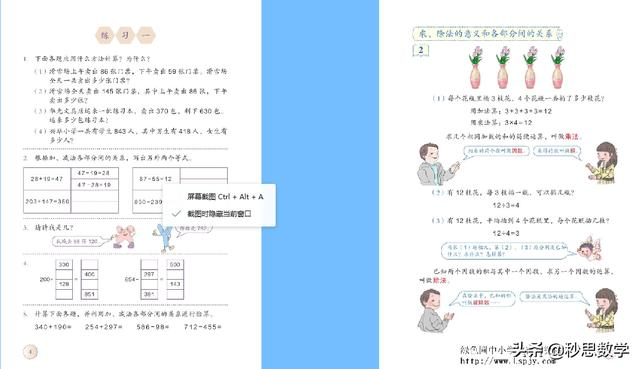# 四年级数学下册第2课时 加、减法的意义和各部分间的关系练习1

2021-01-04 39 ℃1.在括号里填上适当的数。

(　　)+347=500　　27+(　　)=100

573-(　　)=170 (　　)-212=40

(　　)+173=300 (　　)-600=200

2.根据给出的算式填空。

(1)400-165=235 235+(　　)=400 400-(　　)=165

(2)256+244=500 500-(　　)=244 500-(　　)=256

3.计算,并验算。

119-59= 173+169= 257+160= 475-180=

4.滨海实验小学举行花式篮球比赛,明明用了240秒完成了比赛,东东用了215秒完成了比赛。他们一共用了多少秒完成比赛?